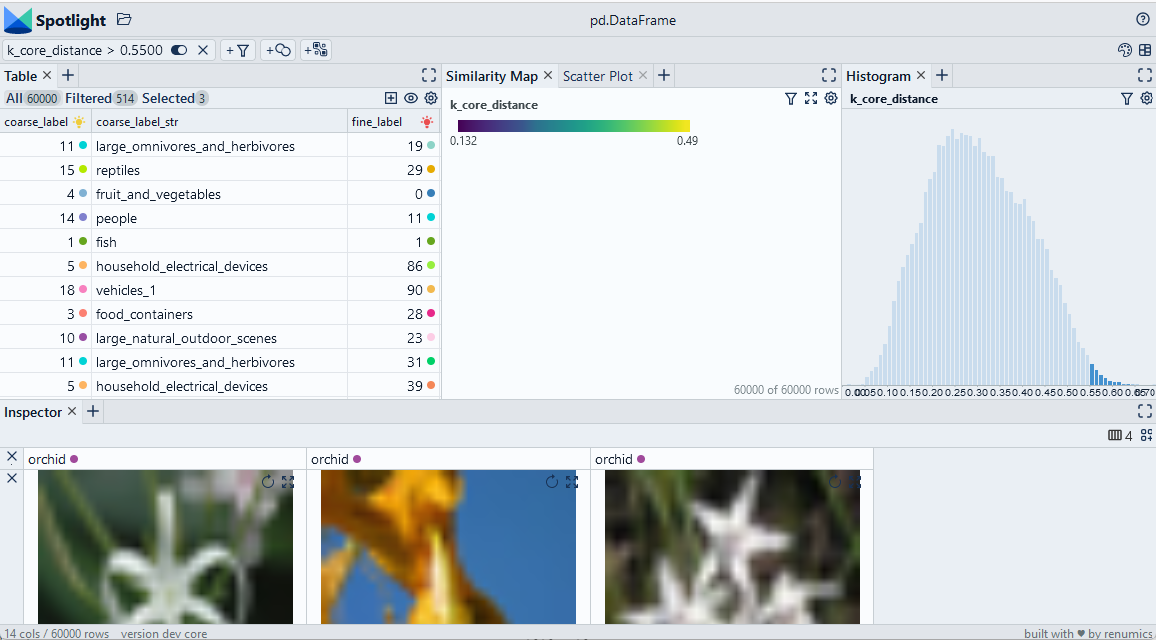🎉 We released Spotlight 1.4.0 check it out

Version: Next

# Detect data drift with the k-core distance

We compute the cosine distance of the k-nearest neighbor in the embedding space for each data sample. This distance can be used to detect outliers/drifted samples. We find a suitable outlier threshold by inspecting the data with Spotlight.

Use Chrome to run Spotlight in Colab. Due to Colab restrictions (e.g. no websocket support), the performance is limited. Run the notebook locally for the full Spotlight experience.• `df['embedding']` contain the embeddings for each data sample## Imports and play as copy-n-paste functions​

# Install dependencies
``#@title Install required packages with PIP!pip install renumics-spotlight datasets``
# Play as copy-n-paste functions
``#@title Play as copy-n-paste functionsfrom sklearn.neighbors import NearestNeighborsimport pandas as pdimport numpy as npimport datasetsfrom renumics import spotlightdef compute_k_core_distances(df, k=8, embedding_name='embedding'):    features = np.stack(df[embedding_name].to_numpy())    neigh = NearestNeighbors(n_neighbors=k, metric='cosine')    neigh.fit(features)    distances, indices = neigh.kneighbors()    df_out=pd.DataFrame()    df_out['k_core_distance']=distances[:,-1]    df_out['k_core_index']=indices[:, -1]    return df_out``

## Step-by-step example on CIFAR-100​

### Load CIFAR-100 from Huggingface hub and convert it to Pandas dataframe​

``dataset = datasets.load_dataset("renumics/cifar100-enriched", split="train")df = dataset.to_pandas()``

### Compute k-nearest neighbor distances​

``df_kcore = compute_k_core_distances(df)df = pd.concat([df, df_kcore], axis=1)``

### Inspect candidates for data drift with Spotlight​

``df_show = df.drop(columns=['embedding', 'probabilities'])layout_url = "https://raw.githubusercontent.com/Renumics/spotlight/playbook_initial_draft/playbook/rookie/drift_kcore.json"response = requests.get(layout_url)layout = spotlight.layout.nodes.Layout(**json.loads(response.text))spotlight.show(df_show, dtype={"image": spotlight.Image, "embedding_reduced": spotlight.Embedding}, layout=layout)``